# 信号完整性 - 传输线 🚧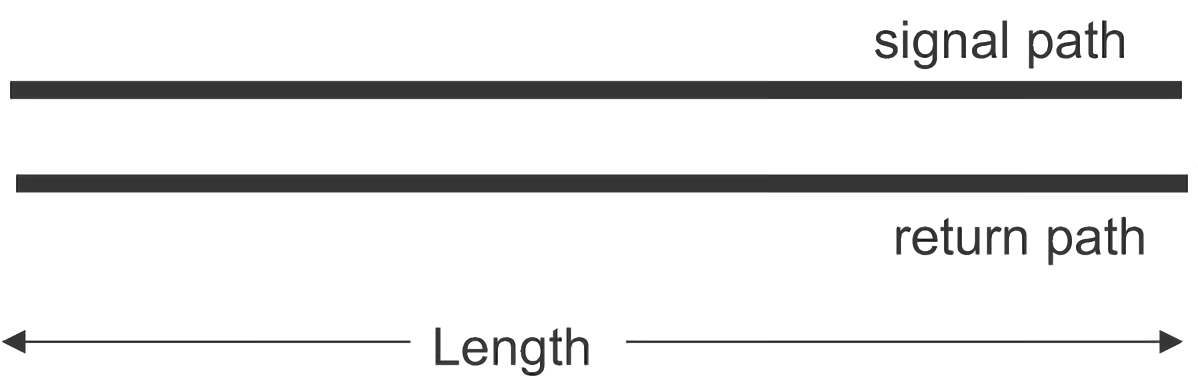## 信号的传输方式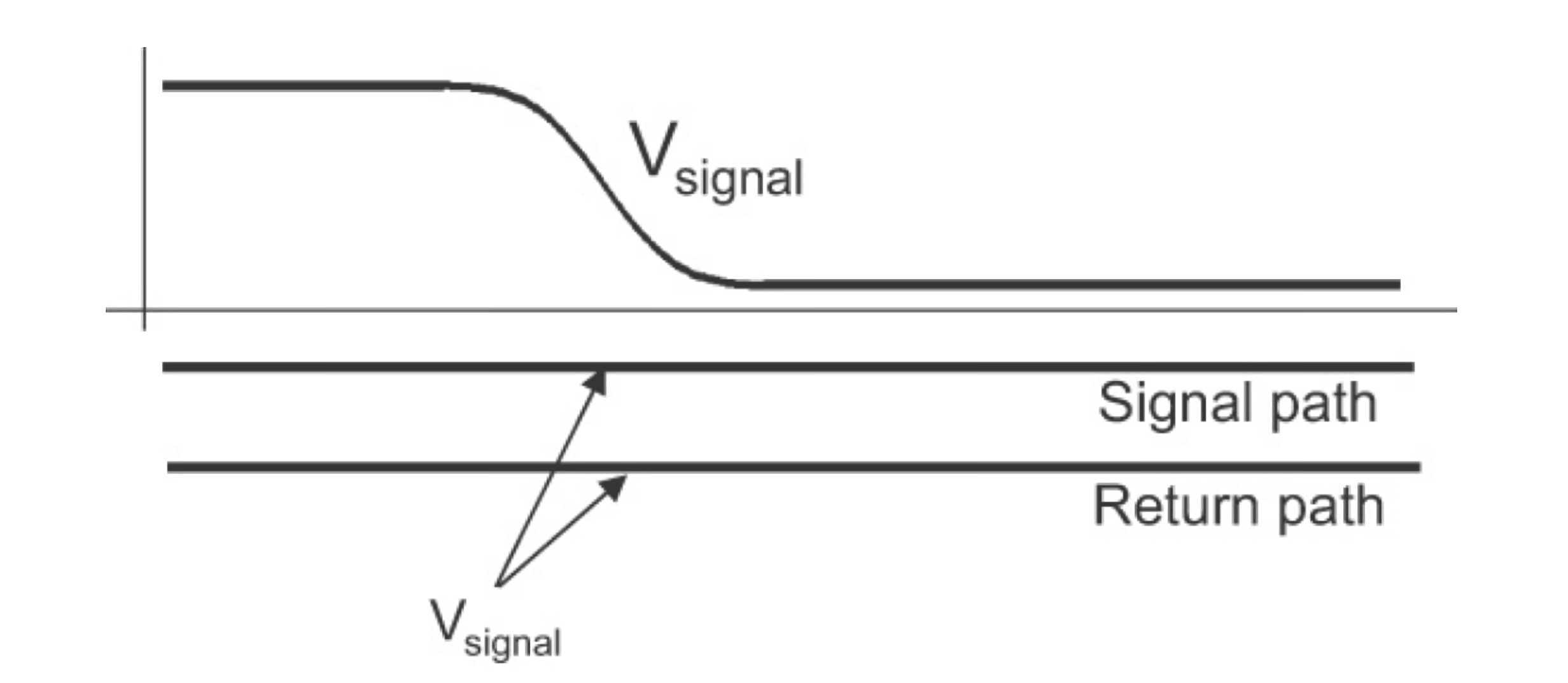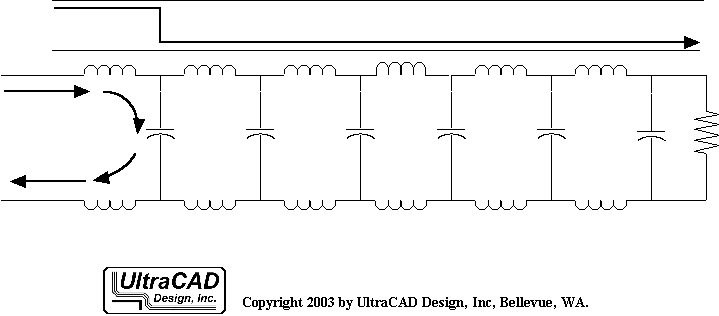## 均匀传输线与平衡传输线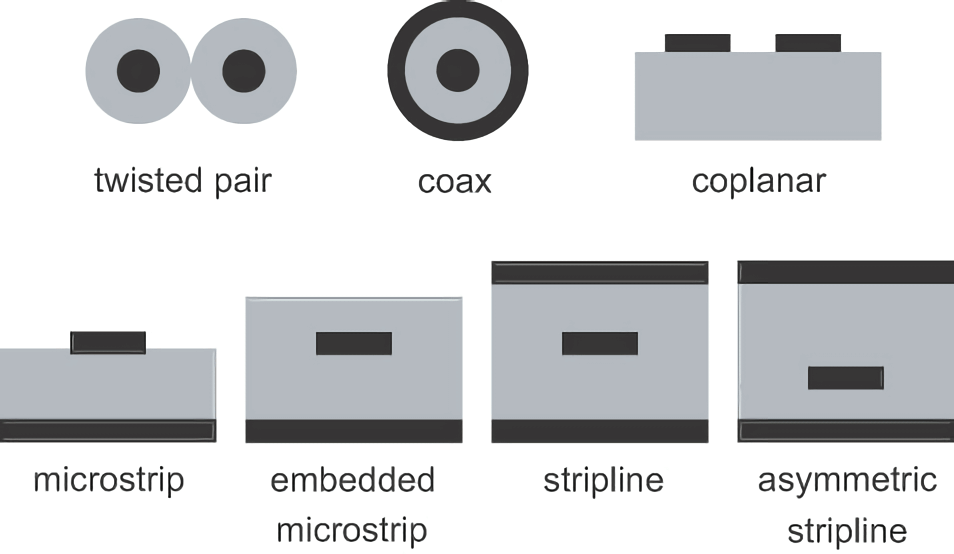## 信号传播的速度

$I=\frac{\Delta Q}{\Delta t}=\frac{qnAv\Delta t}{\Delta t}=qnAv$

$v=\frac{I}{qnA}$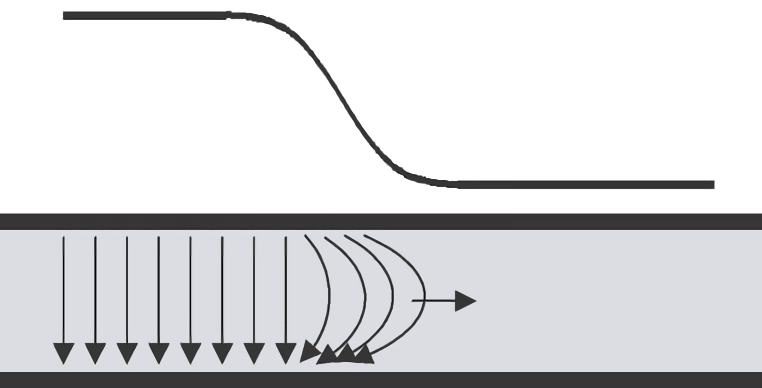$v=\frac{1}{\sqrt{\varepsilon_0\varepsilon_r\mu_0\mu_r}}$

$v=\frac{2.99\times 10^8}{\sqrt{\varepsilon_r}} m/s =\frac{12}{\sqrt{\varepsilon_r}}inch/ns$

$v=\frac{12}{\sqrt{\mu_r}}inch/ns$

## 前沿的空间延伸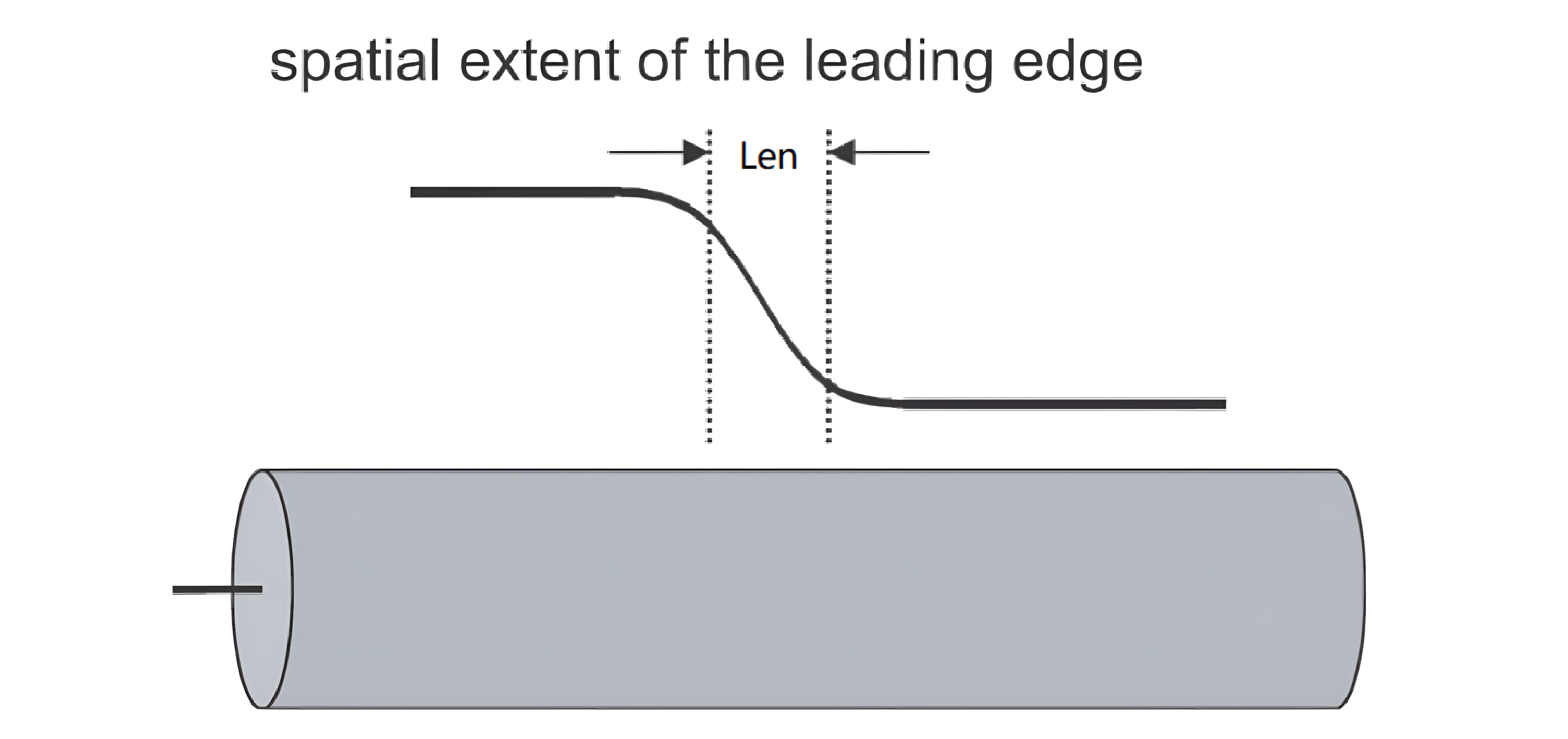$Len=RT\times v$

## 单位长度电容

PCB 上的传输线，如果横截面积不变，那么可以使用集总电容模型，用单位长度电容来表示电容参数，这样总电容就与传输线长度成比例，这样会方便建模。

## 回路电感

$L_{loop}=L_{Ss}+L_{Fs}-2L_{SFm}$

## 瞬态阻抗与特性阻抗 √

$Z_0=\sqrt{\frac{L}{C}}$

## 传输线的驱动与内阻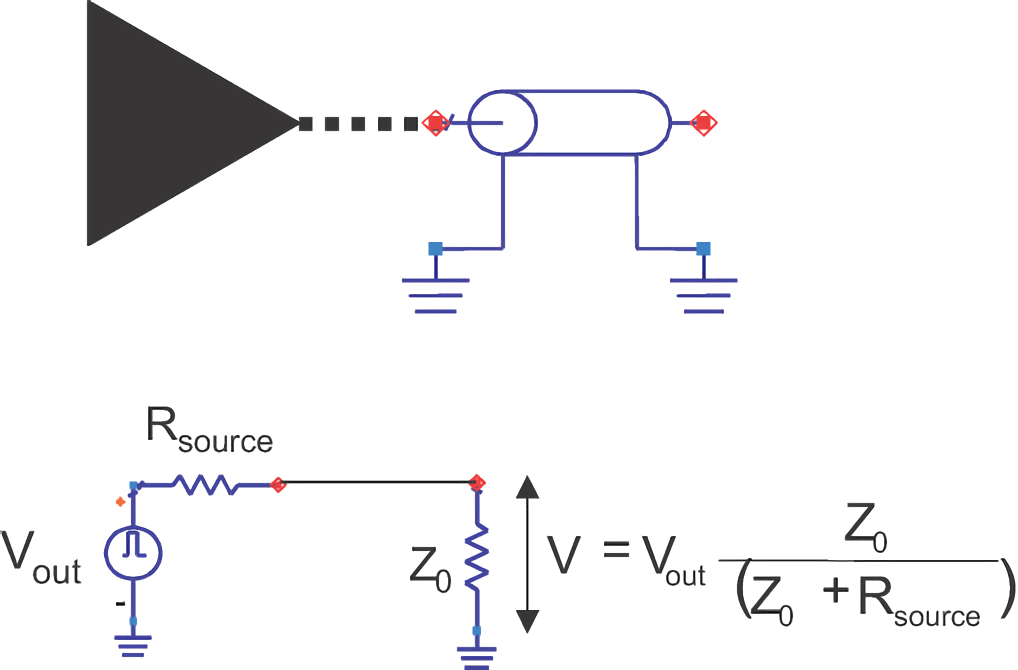## 返回路径与参考平面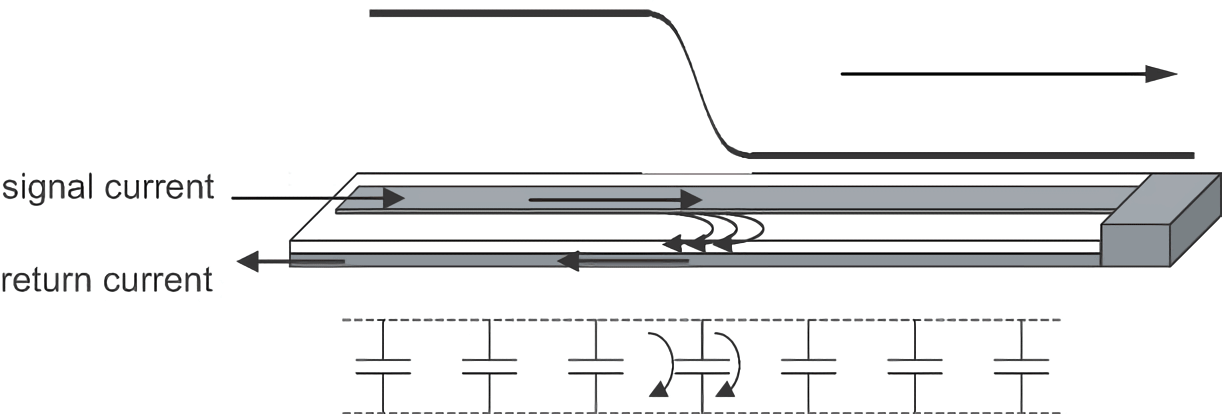## 理想传输线的一阶模型 🚧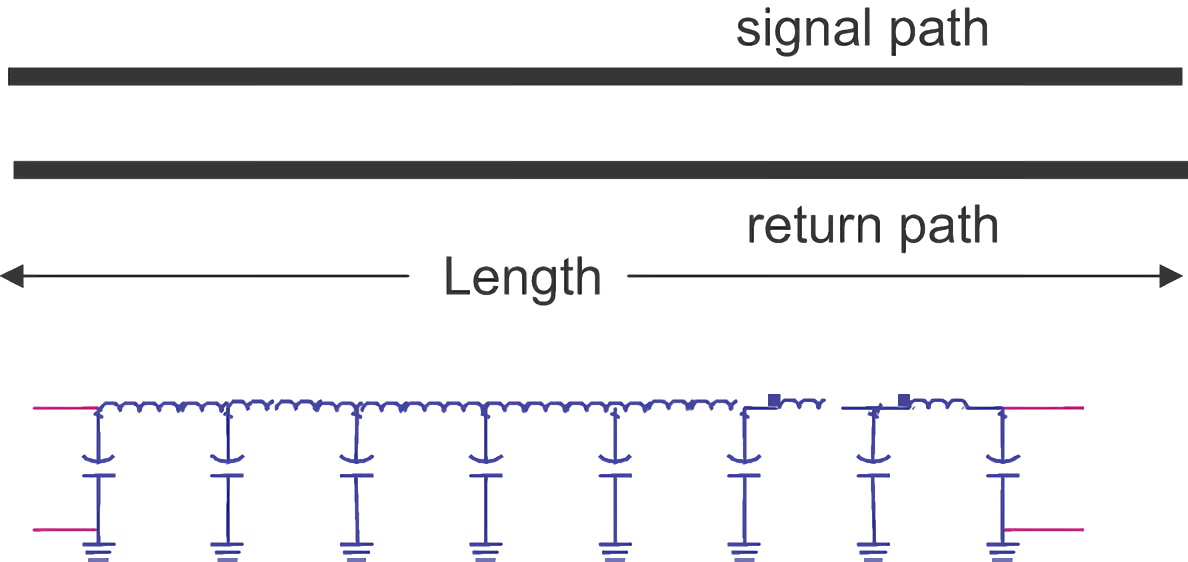$C_{total}=C_L*Len$
$L_{total}=L_L*Len$

$Z_0=\sqrt{\frac{L_L}{C_L}}$
$T_D=\sqrt{C_{total}*L_{total}}=Len*\sqrt{C_L*L_L}$
$v=\frac{Len}{T_D}=\frac{1}{\sqrt{C_L*L_L}}$

$\because v=\frac{c}{\sqrt{\varepsilon_r}}=\frac{Len}{T_D}=\frac{1}{\sqrt{C_L*L_L}}$

## 参考与致谢

• 《信号完整性与电源完整性分析》
• 《信号完整性揭秘 - 于博士 SI 设计手记》